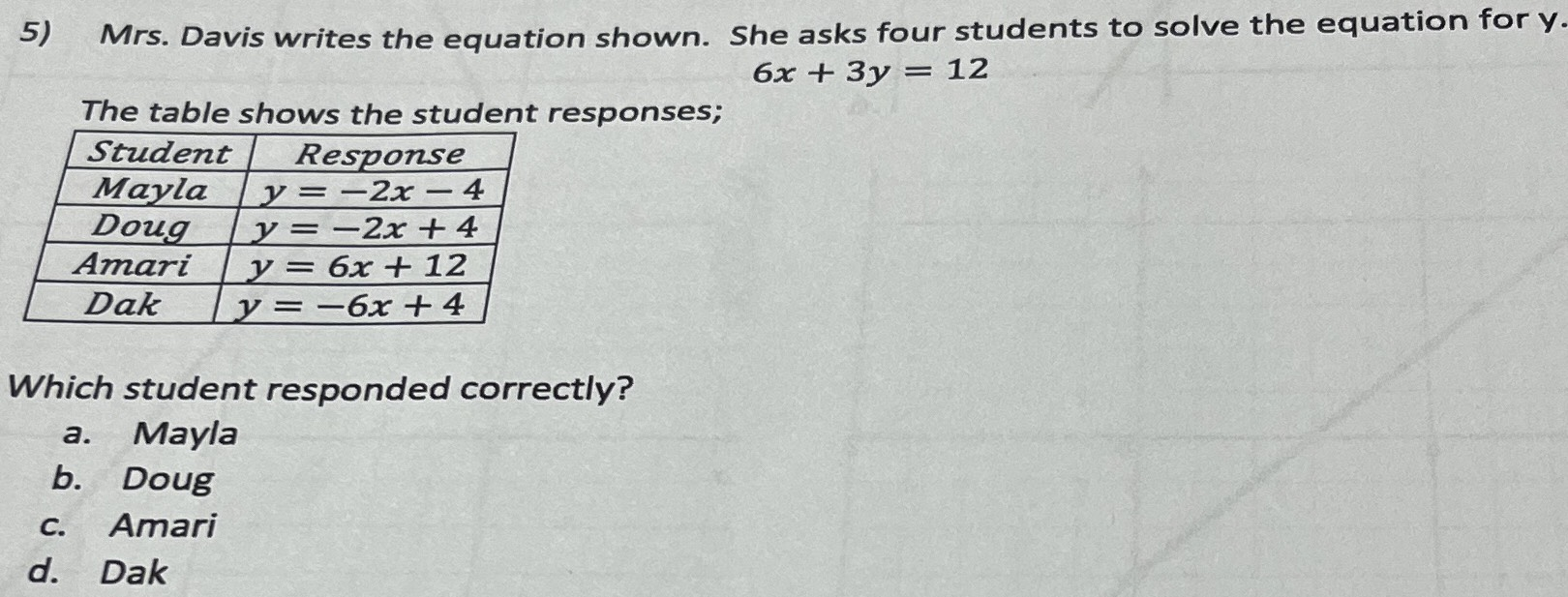### ¿Todavía tienes preguntas de matemáticas?

Pregunte a nuestros tutores expertos
Algebra
Pregunta5) Mrs. Davis writes the equation shown. She asks four students to solve the equation for y.

$$6 x + 3 y = 12$$

The table shows the student responses;

Which student responded correctly?

a. Mayla

b. Doug

c. Amari

d. Dak